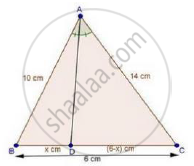Share

# In a δAbc, Ad is the Bisector of ∠A, Meeting Side Bc at D. If Ab = Lo Cm, Ac =14 Cm and Bc =6 Cm, Find Bd and Dc. - CBSE Class 10 - Mathematics

#### Question

In a ΔABC, AD is the bisector of ∠A, meeting side BC at D.

If AB = 10 cm, AC =14 cm and BC =6 cm, find BD and DC.

#### SolutionIn ΔABC, AD is the bisector of ∠A

We know that, the internal bisector of an angle of a triangle divides the opposite side internally in the ratio of the sides containing the angle.

therefore"BD"/"DC"="AB"/"AC"

rArrx/(6-x)=10/14

⇒ 14x = 10(6 – x)

⇒ 24x = 60

rArrx=60/24=5/2=2.5  cm

Since, DC = 6 – x = 6 – 2.5 = 3.5 cm

Hence, BD = 2.5cm, and DC = 3.5 cm

Is there an error in this question or solution?

#### Video TutorialsVIEW ALL 

Solution In a δAbc, Ad is the Bisector of ∠A, Meeting Side Bc at D. If Ab = Lo Cm, Ac =14 Cm and Bc =6 Cm, Find Bd and Dc. Concept: Angle Bisector.
S